NCERT Class 8 Maths Cubes and Cube Roots

# NCERT Class 8 Maths Cubes and Cube Roots

The chapter 7 begins with an introduction to cube roots by citing the very interesting Hardy – Ramanujan Number.Then cubes are explained elaborately and interesting patterns associated with it such as adding consecutive odd numbers, cubes and their prime factors are described.Then the smallest multiple that is a perfect cube is explained briefly.The next topic of discussion is cube roots and the procedure to find the cube roots through prime factorisation method is dealt in detail.The cube root of a cube number is the last topic of discussion.

## Chapter 7 Ex.7.1 Question 1

Which of the following numbers are not perfect cubes?

(i) $$216$$

(ii) $$128$$

(iii) $$1000$$

(iv) $$100$$

(v) $$46656$$

### Solution

What is unknown?

To find the numbers which are not perfect cubes.

Reasoning:

A number is a prefect cube only when each factor in the prime factorization is grouped in triples.

Steps:

(i)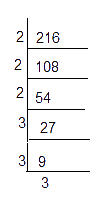\begin{align}216 &= {2 \times 2 \times 2} \times {3 \times 3 \times 3} \\&= {2^3} \times {3^3}\\& ={{(2\times 3)}^{3}} \\& ={{6}^{3}} \\\end{align}

$$\therefore 216$$ is a perfect cube

(ii)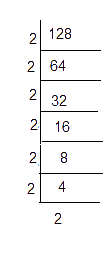\begin{align}128 = 2 \times 2 \times 2 \times 2 \times 2 \times 2 \times 2 \end{align}

$$128 = {2^3} \times {2^3} \times 2$$ one of the $$2$$ is not grouped in triples

$$\therefore \;128$$ is not a perfect cube

(iii)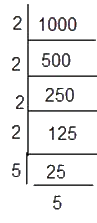\begin{align}1000 &= 2 \times 2 \times 2 \times 5 \times 5 \times 5\\ 1000 &= {2^3} \times {5^3}\end{align}

$$\therefore\;1000$$ is a perfect cube

(iv)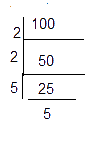\begin{align}100&= 2 \times 2 \times 5 \times 5\\&= {2^2} \times {5^2}\end{align}

Both $$2$$ and $$5$$ are not grouped in triples

$$\therefore \;100$$ is not a perfect cube.

(iv)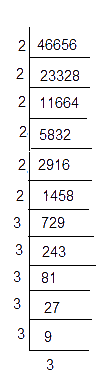\begin{align}46656 &= \left(\begin{array} \ \underline {2 \times 2 \times 2} \times \underline {2 \times 2 \times 2} \times \\ \underline {3 \times 3 \times 3} \times \underline {3 \times 3 \times 3}\end{array} \right) \\ &= {2^3} \times {2^3} \times {3^3} \times {3^3} \end{align}

$$\therefore\;46656$$ is a perfect cube

## Chapter 7 Ex.7.1 Question 2

Find the smallest number by which each of the following numbers must be multiplied to obtain a perfect cube.

(i) $$243$$

(ii) $$256$$

(iii) $$72$$

(iv) $$675$$

(v) $$100$$

### Solution

What is unknown?

To find the smallest number by which the given number must be multiplied to obtain a perfect cube.

Reasoning:

A number is a perfect cube only when each factor in the prime factorization is grouped in triples.Using this concept, the smallest number can be identified.

Steps:

(i)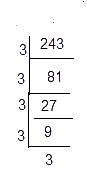\begin{align}243 &= \underline {3 \times 3 \times 3} \times 3 \times 3\\243 &= {3^3} \times 3\end{align}

Here, one of the $$3'\rm{s}$$ is  not a triplet.To make it as a triplet, we need to multiply by $$3$$

In that case,

\begin{align}243 \times 3 &= \underline {3 \times 3 \times 3} \times \underline {3 \times 3 \times 3} \\&= {3^3} \times {3^3} \\&= {9^3} = 729\end{align}

is a perfect cube.

Hence, the smallest natural number by which $$243$$ should be multiplied to make a perfect cube is $$3$$.

(ii)

\begin{align}256 &= \underline {2 \times 2 \times 2} \times \underline {2 \times 2 \times 2}\\& \times 2 \times 2\\256 &= {2^3} \times {2^3} \times {2^2}\end{align}

Here one of the $$2’\rm{s}$$ is not a triplet. To make it as a triplet, we need to multiply by $$2.$$

In that case,

\begin{align}256 \times 2 &= \underline {2 \times 2 \times 2} \times \underline {2 \times 2 \times 2} \\&\times \underline {2 \times 2 \times 2} \\&= {2^3} \times {2^3} \times {2^3} \\&= {8^3} = 512\end{align}

is a perfect cube.

Hence, the smallest natural number by which $$256$$ should be multiplied to make a perfect cube is $$2$$.

(iii)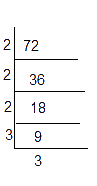\begin{align}72 &= \underline {2 \times 2 \times 2} \times 3 \times 3\\72 &= {2^3} \times {3^2}\end{align}

Here,on of the $$3$$'s is not a triplet. To make it as a triplet, we need to multiply by $$3.$$

In that case,

\begin{align}72 \times 3 &= \underline {2 \times 2 \times 2} \times \underline {3 \times 3 \times 3} \\&= {2^3} \times {3^3} \\&= {6^3} = 216\end{align}

is a perfect cube.

Hence, the smallest natural number by which 72 should be multiplied to make a perfect cube is 3.

(iv)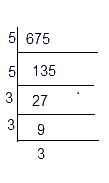\begin{align}675 &= 5 \times 5 \times \underline {3 \times 3 \times 3} \\675 &= {5^2} \times {3^3}\end{align}

Here, one of the $$5$$'s is not a triplet. To make it as a triplet, we need to multiply by $$5.$$

In that case,

\begin{align}675 &= \underline {5 \times 5 \times 5} \times \underline {3 \times 3 \times 3} \\&= {5^3} \times {3^3} \\&= {15^3} = 3375\end{align}

is a perfect cube.

Hence, the smallest natural number by which $$675$$ should be multiplied to make a perfect cube is $$5$$.

(v)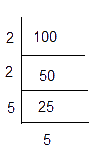\begin{align}100 &= 2 \times 2 \times 5 \times 5\\100 &= {2^2} \times {5^2}\end{align}

Here both the prime factors are not triplets. To make them triplets we need to multiply by one more  $$2$$ and $$5.$$

In that case,

\begin{align}100 &=\, \underline {2 \times 2 \times 2} \times \underline {5 \times 5 \times 5} \\&= {2^3} \times {5^3} \\&= {10^3} = 1000\end{align}

is a perfect cube.

Hence, the smallest natural number by which 100 should be multiplied to make a perfect cube is $$2 \times 5 = 10.$$

## Chapter 7 Ex.7.1 Question 3

Find the smallest number by which each of the following numbers must be divided to obtain a perfect cube.

(i) $$81$$

(ii) $$128$$

(iii) $$135$$

(iv) $$192$$

(v) $$704$$

### Solution

What is unknown?

To find the smallest number by which a given number must be divided to obtain a perfect cube.

Reasoning:

A number is a perfect cube only when each factor in the prime factorization is grouped in triples. Using this concept smallest number to be multiplied can be obtained.

(i)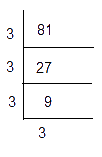\begin{align}81 &= \underline {3 \times 3 \times 3} \times 3\\81 &= {3^3} \times 3\end{align}

Here, the prime factor $$3$$ is not present as triples.

Hence, we divide by $$81$$ by $$3,$$ so that the obtained number becomes a perfect cube.

Thus,

\begin{align}81 \div 3 = 27 = {3^3}\end{align} is a perfect cube.

Hence the smallest number by which $$81$$ should be divided to make a perfect cube is $$3$$.

(ii)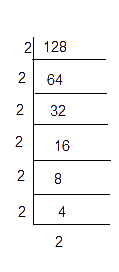\begin{align}128& = \underline {2 \times 2 \times 2} \times \underline {2 \times 2 \times 2} \times 2\\128 &= {2^3} \times {2^3} \times 2\end{align}

Here,the prime factors $$2$$ is not present as triples.

Hence, we divide $$128$$ by $$2,$$ so that the obtained number becomes a perfect cube.

\begin{align}128 \div 2 = 64 = {2^3} \times {2^3} = {4^3}\end{align} is a perfect cube.

Hence the smallest number by which $$128$$ should be divided to make a perfect cube is $$2$$.

(iii)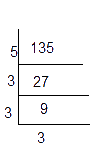\begin{align}135 &= 5 \times \underline {3 \times 3 \times 3} \\135 &= {5^1} \times {3^3}\end{align}

Here, the prime factors $$5$$ is not present as triples.

Hence, we divide $$135$$ by $$5,$$ so that the obtained number becomes a perfect cube.

\begin{align}135 \div 5 = 27 = {3^3}\end{align} is a perfect cube.

Hence the smallest number by which $$135$$ should be divided to make a perfect cube is $$5$$.

(iv)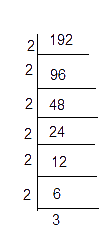\begin{align}192 &= \underline {2 \times 2 \times 2} \times \underline {2 \times 2 \times 2} \times 3\\192 &= {2^3} \times {2^3} \times 3\end{align}

Here,the prime factors $$3$$ is not present as triples.

Hence, we divide $$192$$  by $$3,$$ so that the obtained number becomes a perfect cube.

\begin{align}192 \div 3 = 64 = {2^3} \times {2^3} = {4^3}\end{align} is a perfect cube.

Hence the smallest number by which $$192$$ should be divided to make a perfect cube is $$3$$.

(v)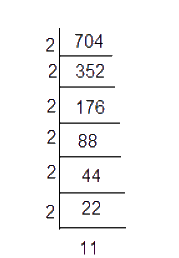\begin{align}704 &= \underline {2 \times 2 \times 2} \times \underline {2 \times 2 \times 2} \times 11\\704 &= {2^3} \times {2^3} \times 11\end{align}

Here, the prime factors $$11$$ is not present as triples.

Hence, we divide $$704$$ by $$11,$$ so that the obtained number becomes a perfect cube.

\begin{align}704 \div 11 = 64 = {2^3} \times {2^3} = {4^3}\end{align} is a perfect cube.

Hence the smallest number by which $$704$$ should be divided to make a perfect cube is $$11$$.

## Chapter 7 Ex.7.1 Question 4

Parikshit makes a cuboid of plasticine of sides $$5 \,\rm{cm},$$ $$2 \,\rm{cm,}$$ $$5\, \rm{cm.}$$ How many such cuboids will he need to form a cube?

### Solution

What is known?

Dimensions of cuboid $$5\;{\rm{cm}}\, \times\,2\;{\rm{cm}}\,\times \,5\;\rm{cm}.$$

What is unknown?

To find out the number of cuboids to form a cube.

Reasoning:

Number of cuboids required

\begin{align}=\frac{{{\text{Volume of cube}}}}{{{\text{Volume of cuboid}}}}\end{align}

Steps:

Volume of cuboid

\begin{align}&={\rm{length}}\! \times\! {\rm{breadth}} \\&\times {\rm{height}}\\ &= 5 \times 2 \times 5\\&= {5^2} \times {2^1}{\rm{c}}{{\rm{m}}^3}\end{align}

To make the volume of cuboid as a cube number we need to multiply it by $$5 \times 2 \times 2$$

\begin{align}&\text {Newly formed cube}\\&= {5^2} \times {2^1} \times 5\; \times 2 \times 2\\&= {5^3} \times {2^3}{\rm{c}}{{\rm{m}}^3}\end{align}

\begin{align}&\text {Number of cuboids required} \\&=\frac{5^{3} \times 2^{3}}{5^{2} \times 2}\\&=\frac{5 \times \not5 \times \not5 \times \not2 \times 2 \times 2}{\not 5 \times \not 5 \times \not2}\end{align}

$$\therefore \;$$ Number of cuboids required to make a cube $$=$$ \begin{align}5\times 2\times 2=20\end{align}

$$20$$ cuboids required.

Cubes and Cube Roots | NCERT Solutions
Instant doubt clearing with Cuemath Advanced Math Program